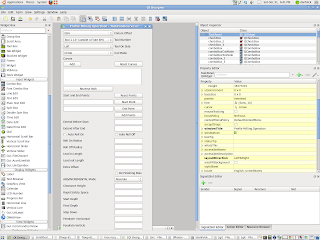## Saturday, December 31, 2011

### Using QtDesigner!!!!I'm starting to get the hang of using QtDesigner for making pyQt Dialogs with FreeCAD/HeeksCNC. I love it!!!!

### A little progress report

I'm still learning the internals of FreeCAD. I helped the main project a little by creating a lowly 'Point' class, which Yorik integrated into the Draft workbench. Doing this helped me get familiar with the source. Having this object class will also be useful later on for CAM work (start and end points for profiles etc).
I have also made some scripts for extracting libarea 'curve' elements from objects in the FreeCAD document. Right now, I can click on edges of solids or lines and arcs in Sketches or Draft elements and then run a macro to produce some python code that can be pasted into a HeeksCNC python script for producing g-code.
The resulting code looks like this:

curve = area.Curve()
#open path
curve.append(area.Point(62.244881,-33.935955))
curve.append(area.Vertex(-1 , area.Point( 57.129109, -39.051727), area.Point(57.129109, -33.935955)))
curve.append(area.Point( -56.043546, -39.051727))
curve.append(area.Vertex(-1 , area.Point( -64.821892, -30.273381), area.Point(-56.043546, -30.273381)))

If you've ever used HeeksCNC, this code should look familiar.
I can take this code and paste it into a script such as this one:

import sys
sys.path.insert(0,'/usr/lib/heekscnc/')
import math
import area
area.set_units(1)
import kurve_funcs
from nc.nc import *
import nc.emc2b

output('/home/danfalck/Documents/freecad/curve_output/test.tap')
program_begin(123, 'Test program')
absolute()
metric()
flush_nc()

set_plane(0)

workplane(1)

#(4.7752 mm Carbide End Mill)
tool_defn( id=4, name='4.7752 mm Carbide End Mill', radius=2.3876, length=23.876, gradient=-0.1)
tool_diameter = float(4.7752)
cutting_edge_angle = float(0)

#path/geometry section
curve = area.Curve()
curve.append(area.Point(62.244881,-33.935955))
curve.append(area.Vertex(-1 , area.Point( 57.129109, -39.051727), area.Point(57.129109, -33.935955)))
curve.append(area.Point( -56.043546, -39.051727))
curve.append(area.Vertex(-1 , area.Point( -64.821892, -30.273381), area.Point(-56.043546, -30.273381)))
#end of path/geometry section

#program action:
comment('tool change to 4.7752 mm Carbide End Mill')
tool_change( id=4)
spindle(7000)
feedrate_hv(840, 100)
clearance = float(5)
rapid_safety_space = float(2)
start_depth = float(0)
step_down = float(2)
final_depth = float(-10)
roll_radius = float(5.0)
offset_extra = 0
roll_on = 'auto'
roll_off = 'auto'
extend_at_start= 0
extend_at_end= 0
lead_in_line_len= 5.0
lead_out_line_len= 5.0
kurve_funcs.profile(curve, 'left', tool_diameter/2, offset_extra, roll_radius, roll_on, roll_off, rapid_safety_space, clearance, start_depth, step_down, final_depth,extend_at_start,extend_at_end,lead_in_line_len,lead_out_line_len )

absolute()

program_end()

The resulting gcode might give something like this (backplotted in FreeCAD):